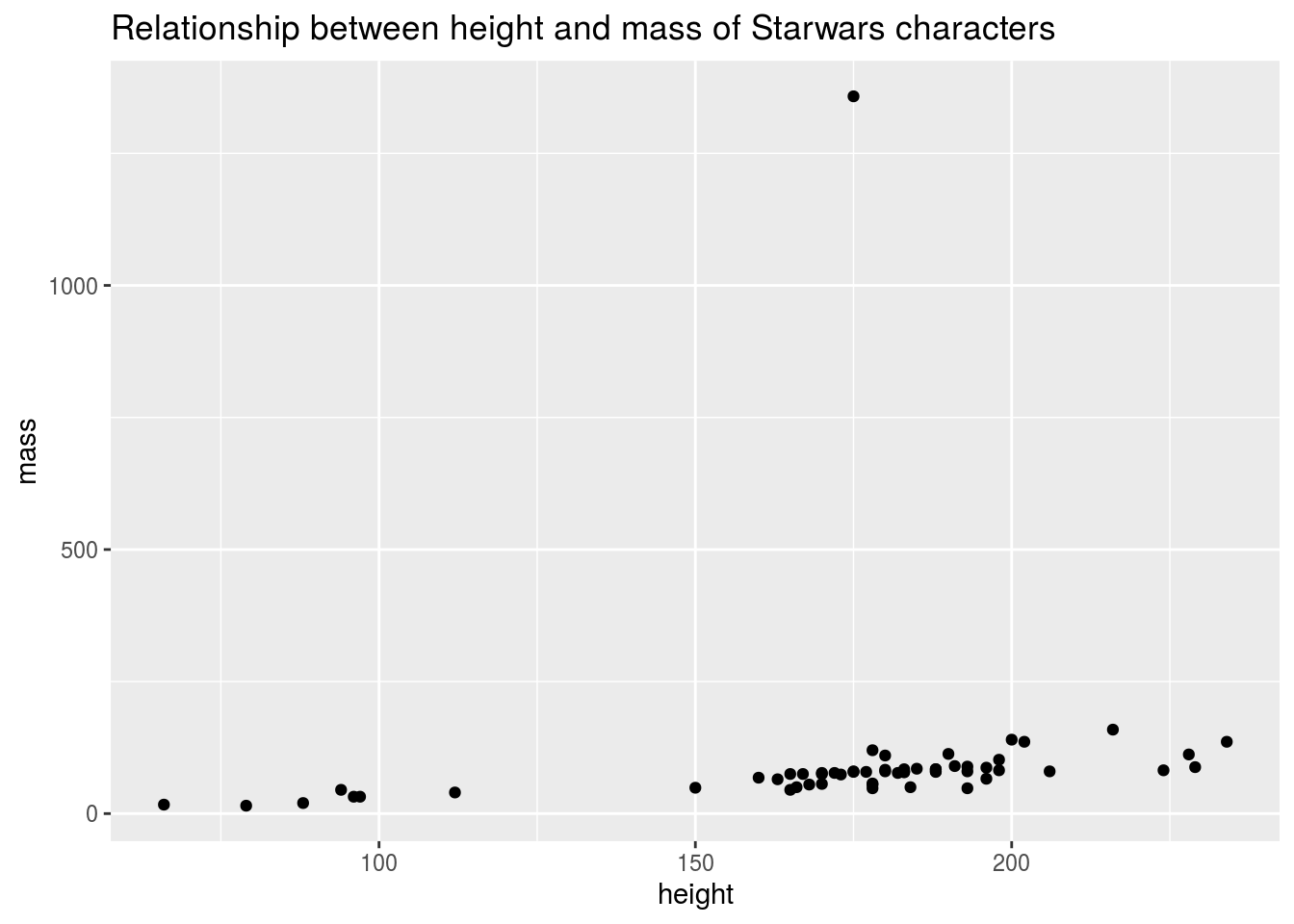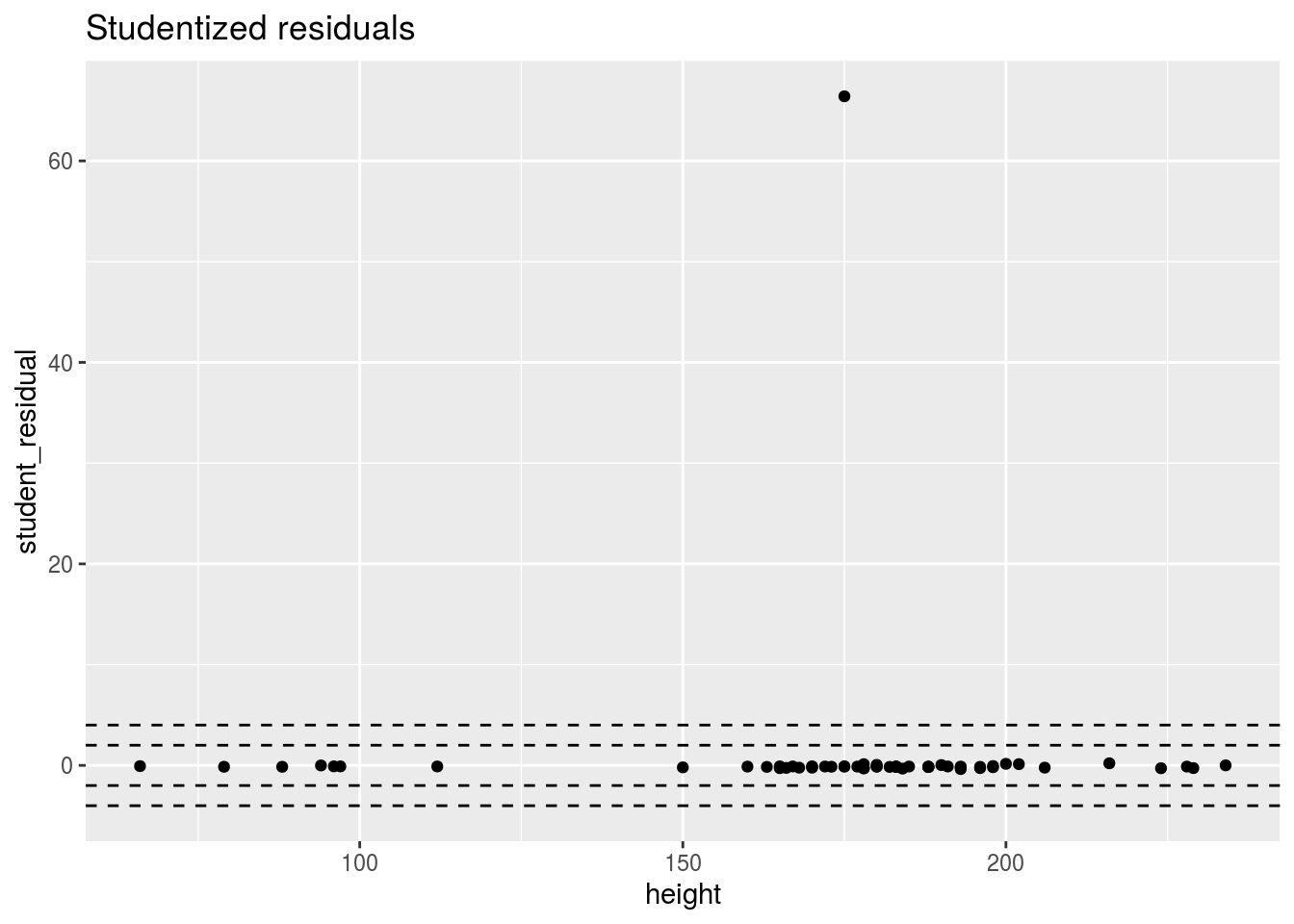library(tidyverse)
starwars_filtered <- starwars %>%
filter(!is.na(mass), !is.na(height))

## Create a scatterplot of mass and height in the starwars dataset

ggplot(data = starwars_filtered, aes(x = height, y = mass)) +
geom_point() +
labs(title = "Relationship between height and mass of Starwars characters")## Fit a model of mass ~ height using the starwars dataset

model <- lm(mass ~ height, starwars_filtered)
model
##
## Call:
## lm(formula = mass ~ height, data = starwars_filtered)
##
## Coefficients:
## (Intercept)       height
##    -13.8103       0.6386

## Calculate the studentized residuals and add them to the starwars dataset

starwars_filtered <- starwars_filtered %>%
mutate(student_residual = rstudent(model))

## Create a plot of the studentized residuals on the y-axis and height on the x-axis

ggplot(starwars_filtered, aes(height, student_residual)) +
geom_point() +
geom_hline(yintercept = c(-4, -2, 2, 4), lty = 2) +
labs(title = "Studentized residuals")## Fit the same regression model (mass ~ height) with and without the “unusual” point

starwars %>%
filter(name != "Jabba Desilijic Tiure") %>%
lm(mass ~ height, data = .)
##
## Call:
## lm(formula = mass ~ height, data = .)
##
## Coefficients:
## (Intercept)       height
##    -32.5408       0.6214